# Uniswap Labs Blog

A Primer on Uniswap v3 Math Part 2: Stay Awake by Reading it Aloud

June 26, 2023

_By: Austin Adams, Sara Reynolds, Kirill Naumov, and Rachel Eichenberger[^1] _

[^1]: Austin is a researcher and Sara is a Protocol Engineer at Uniswap Labs. Kirill is an undergraduate student at the Wharton School and head of governance at FranklinDAO. Rachel received a Uniswap Grant to support the developer community.

Liquidity math can get quite overwhelming. This piece will provide a detailed explanation with concepts, math, and code to help traders, researchers, and liquidity providers better understand all things liquidity-related in Uniswap v3 and v4. (The liquidity math in Uniswap v4 is the same as v3.)

In the first part of this series, A primer on Uniswap v3 math: As easy as 1, 2, v3, we answered some common math questions around Q Notation, calculating exchange rates, and ticks.

In part two, we're addressing another set of questions around:

• Working with virtual liquidity
• Calculating LP holdings
• Calculating uncollected fees in a position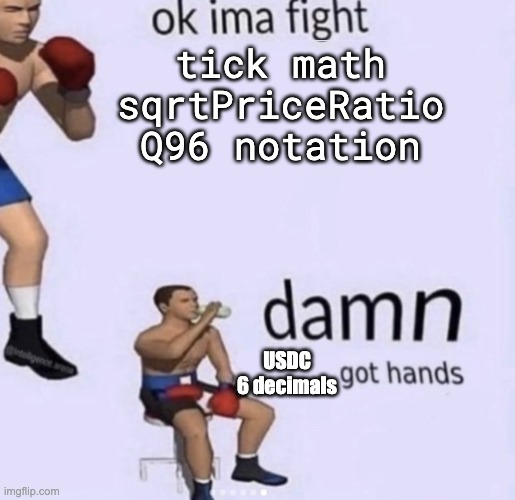Source

# Working with Virtual Liquidity

If you're familiar with how automatic market makers (AMMs) work, you might get a bit confused when first reading about Uniswap v3. In Uniswap v3, the concept of liquidity works slightly differently than in other AMMs. Common challenges involve converting liquidity into USD value and interpreting the mechanics behind liquidity.

In Uniswap v2, liquidity was represented with ERC-20 LP token and was spread evenly across the entire xy=k price range. In v3, liquidity providers can concentrate their liquidity, effectively moving liquidity from the edges of the price range into a price range that a given asset usually trades within. Uniswap v3 makes LPing much more capital efficient. By concentrating their position within a price range, LPs can earn more fees on the same amount of capital than in v2.

Below is an infographic of how liquidity changes depending on the chosen price range. As liquidity providers shrink their range, the same amount of capital is split among fewer ticks.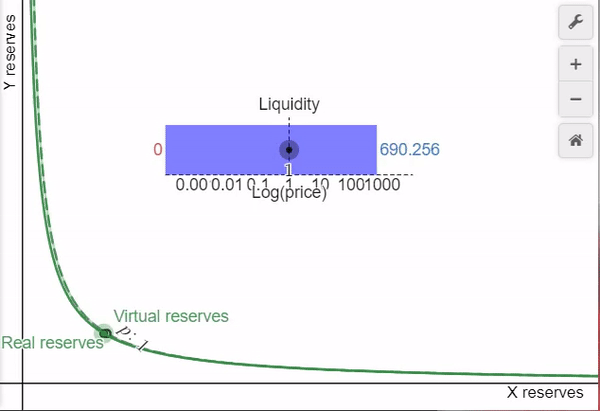For example, for stablecoin pools such as DAI/USDC, LPs can concentrate their capital around the 0.999 to 1.001 range as these two tokens commonly trade within that range. In v2, a $1 million position would be distributed across the entire xy=k curve and users would only be able to trade 200 USDC for DAI before the price drops down to 0.999. Alternatively, if the$1 million of liquidity is within the ticks[^2] that represent the 0.999 to 1.001 range, users would be able to trade 500,000 USDC for DAI before the price moves by the same amount.

[^2]: These prices are most closely represented by tick -8 and tick 8, which equate directly to and $1.0001^8 \approxeq 1.0008$. To make the example clearer, we round the excess digits.

When we talk about liquidity in these pools, we really mean virtual liquidity. When we concentrate liquidity within a range, we construct a virtual xy=k price curve that works exactly like v2, but within the specified price range. This virtual curve is designed to ensure that the amount of assets (represented by real x and y) traded as the price approaches either bound of the range is equal to the real liquidity that has been deposited into the range. Liquidity is constant between ticks, similar to k in Uniswap v2's xy=k model, and can only be adjusted by depositing or withdrawing liquidity from the protocol.

We can calculate liquidity as the square root of the multiple virtual reserves within the range. It's stored as a square root for gas efficiency.

$L = \sqrt{x_{ virtual } \ast y_{ virtual }}$

We can calculate liquidity using the real reserves deposited in the Protocol. Both of these formulas should give the same result. Here $p_l$ and $p_u$ are the lower and upper bounds of the range, and $p'$ is the current price.

$L_x = x\frac{\sqrt{p}'\sqrt{p_b}}{\sqrt{p_b}-\sqrt{p}'}$ $L_y = \frac{y}{\sqrt{p}' - \sqrt{p_a}}$

By accessing deployed Uniswap smart contracts, you can query the net change in liquidity at each tick by calling the ticks() function and cumulatively summing liquidityNet for all initialized ticks -887272 to 887272. You can alternatively use the liquidity() function on the UniswapV3Pool contract to get the current in range liquidity, and remove liquidityNet for each tick below the current tick and add for each tick above the current tick to derive the liquidity for the desired tick. These are fundamentally equal.[^3]

[^2]: Please note, there is numeric instability in the Uniswap v3 subgraph. This can cause some negative values in liquidity, but this is fundamentally impossible in the actual v3 contracts. To best account for this, we recommend setting liquidity to 0 if it is negative.

# Calculating current holdings

## Background

One of the most important things for an LP is calculating the current holdings of their position(s). As the sqrtPriceX96 (price) of the pool changes, the token holdings in each v3 liquidity position could rebalance[^4]. The price of the pool is moved by users trading against the pool’s liquidity, thus shifting the balance in the holdings of LPs. If the liquidity is not in range, then the LP’s position is fully denominated in one of the two tokens of the pool.

[^4]: Note that only in range positions will rebalance

Let’s first explore the process of determining whether a position is in range and then calculate current holdings.

There are four important values needed to calculate your current holdings in Uniswap v3. Everything else can be derived from these values.

### Required inputs to calculate holdings

| Name | Notation | Found in | Function to call | | ---------- | ---------- | ---------- | ---------- | | liquidity | $\ell$ | NonfungiblePositionManager | positions(tokenId) | | tickUpper | $i_u$ | NonfungiblePositionManager | positions(tokenId) | | tickLower | $i_l$ | NonfungiblePositionManager | positions(tokenId) | | sqrtPriceX96 | $\sqrt{P}$ | UniswapV3Pool | slot0() |

### In range positions

Uniswap v3’s concentrated liquidity feature allows LPs to provide liquidity within a set price range, letting them use their capital efficiently. A position is said to be in range when the current price is within the parameters set by the LP when the position was created.

We can read data from the Uniswap smart contracts to calculate when a position is in range using the current tick from sqrtPriceX96 or by pulling the current tick ( $i_c$ ) from the pool contract by querying slot0. The current tick is not strictly necessary for any other calculation and can be derived from the sqrtPriceX96. If you want a refresher on ticks and pricing, please read our part 1 here.

$\frac{\sqrt{P}}{2^{96}} = 1.0001^{i_c}$ $\frac{ \log \frac{\sqrt{P}}{2^{96}}}{ \log 1.0001 } = i_c$

The position is in range if:

• The current tick is greater than or equal to your tickLower
• The current tick is strictly less than your tickUpper
$i_l \leq i_c < i_u$

## How to calculate current holdings?

There are two different methods to calculate the amount of tokens held in a position:

• When a position is in range
• When a position is out of range

The equations are different, but both require us to calculate sqrtRatioL and sqrtRatioU, which represent the upper and lower bounds of a position.

sqrtRatioL or $\sqrt{p_l}$ is the square rate of the price at tickLower: $\sqrt{1.0001^{i_l}}$

sqrtRatioU or $\sqrt{p_u}$ is the square rate of the price at tickUpper: $\sqrt{1.0001^{i_u}}$

### Calculating holdings if the position is in range

If we are in range, we need to calculate the sqrtPrice, $\sqrt{p}'$, This converts the price from a fixed point number (Q notation) to floating point (decimals). You can read more about this in part 1.

$\sqrt{p}' = \sqrt{P} / 2^{96}$

We can then use $\sqrt{p}'$ to calculate the token holdings within the position.

$token_0 = \ell \frac{\sqrt{p_u} - \sqrt{p}'}{\sqrt{p}'\sqrt{p_u}}$ $token_1 = \ell (\sqrt{p}' - \sqrt{p_l})$

### Calculating holdings if the position is out of range

The price of a pool will change as swappers trade in and out. In Uniswap v3, if the sqrtPrice moves outside of an LP’s defined price range, your position will be completely in one token or the other. This requires a new set of equations.

Your position can be out of range for one of two reasons: the price is lower than the position’s lower bound, $\sqrt{p_l}$, or the price is above the upper bound, $\sqrt{p_u}$.

If $\sqrt{p}' \le \sqrt{p_l}$ then you can calculate your holdings via:

$token_0 = \ell \frac{\sqrt{p_u} - \sqrt{p_l}}{\sqrt{p_l}\sqrt{p_u}}$ $token_1 = 0$

If $\sqrt{p_u} \leq \sqrt{p}'$ then you can calculate your holdings via:

$token_0 = 0$ $token_1 = \ell (\sqrt{p_u} - \sqrt{p_l})$

For an in-depth explanation of these formulas, check out this PDF by Elsts. It features slightly different notation, but the fundamental ideas are the same!

## Example position

Let’s go over an example Uniswap v3 position - position 37[^5].

[^5]: While the Uniswap Interface refers to the token pair as ETH/USDC, it is actually WETH/USDC. This is because WETH is an ERC-20 version of ETH.

| Name | Notation | Found in | Value at the time of writing | | ---------- | ---------- | ---------- | ---------- | | liquidity | $\ell$ | NonfungiblePositionManager | 10860507277202 | | tickUpper | $i_u$ | NonfungiblePositionManager | 193380 | | tickLower | $i_l$ | NonfungiblePositionManager | 192180 | | sqrtPriceX96 | $\sqrt{P}$ | NonfungiblePositionManager | 1906627091097897970122208862883908 |

First, let’s figure out if the position is in range. To do this, we need to calculate the current tick of the pool using sqrtPriceX96. We can do that by using the formula mentioned previously

$\frac{ \log \frac{\sqrt{P}}{2^{96}}}{ \log 1.0001 } = i_c$ $\frac{ \log \frac{ 1906627091097897970122208862883908 }{2^{96}}}{ \log 1.0001 } = i_c$ $201780.378 = i_c$

From this, we can check whether the price of a pool is within range.

$i_l ≤ i_c < i_u$

Currently, the condition is not met. We are currently out of range, as the price is higher than the top of our range. This informs which route we take to calculate holdings.

$i_c > i_u$ $201780.378 > 193380$

Now we need to calculate the current sqrtRatioL and sqrtRatioU.

$sqrtRatioL = \sqrt{p_l} = \sqrt{1.0001^{i_l}} = \sqrt{1.0001^{192180}} = 14891.1087$ $sqrtRatioU = \sqrt{p_u} = \sqrt{1.0001^{i_u}} = \sqrt{1.0001^{193380}} = 15811.876$

We know that $\sqrt{p}' > \sqrt{p_u}$ , because $i_c > i_l$.

$token_0 = 0$ $token_1 = \ell (\sqrt{p_u} - \sqrt{p_l}) = 10860507277202(15811.876-14891.1087) = 9.99999996\ast10^{15}$

$token_1$ is the WETH token, which has 18 decimals. We can adjust the raw $token_1$ value to get the adjusted $token_1$ value of

$adjToken_1 = 9.99999996 \ast \frac{10^{15}}{10^{18}} = 0.009999$

Here is a current screenshot from the Uniswap Interface, showing that we calculated the correct amount of tokens!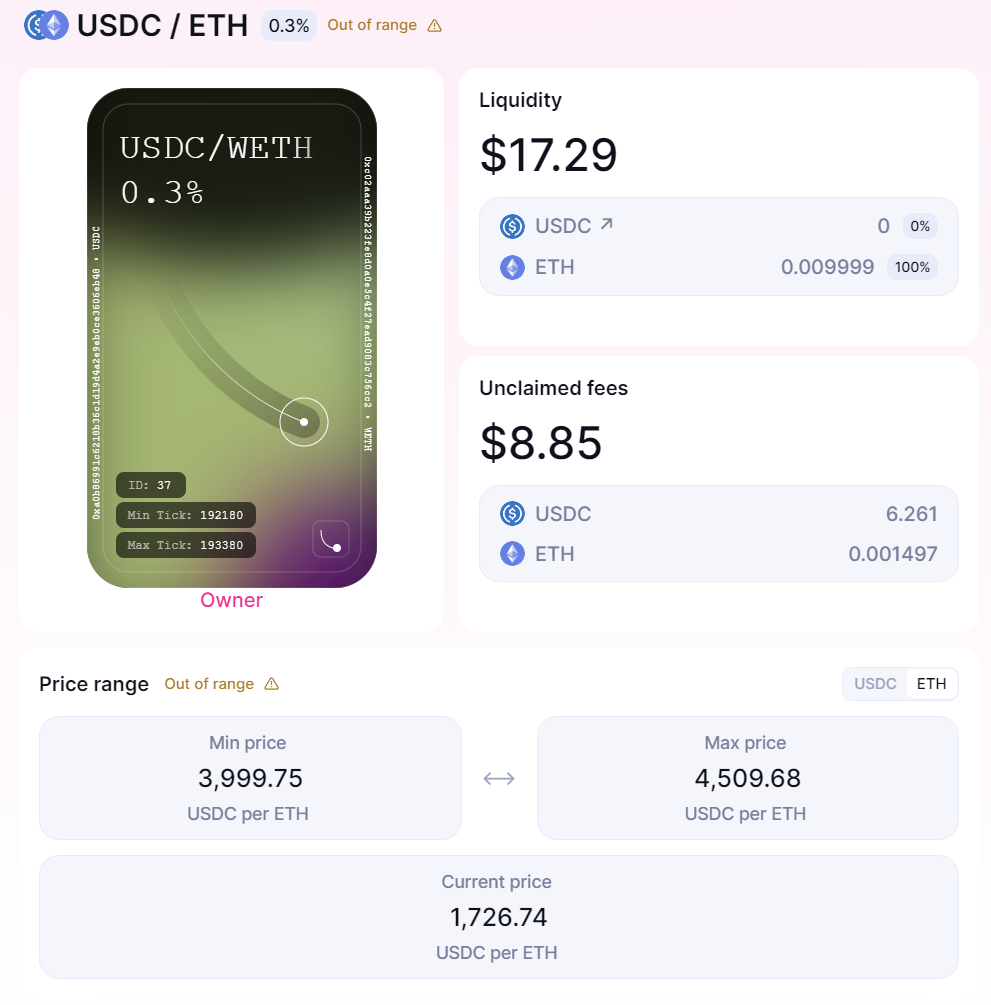## Code example

const Q96 = JSBI.exponentiate(JSBI.BigInt(2), JSBI.BigInt(96));

function getTickAtSqrtPrice(sqrtPriceX96){
let tick = Math.floor(Math.log((sqrtPriceX96/Q96)**2)/Math.log(1.0001));
return tick;
}

async function getTokenAmounts(liquidity,sqrtPriceX96,tickLow,tickHigh,Decimal0,Decimal1){
let sqrtRatioA = Math.sqrt(1.0001**tickLow);
let sqrtRatioB = Math.sqrt(1.0001**tickHigh);
let currentTick = getTickAtSqrtPrice(sqrtPriceX96);
let sqrtPrice = sqrtPriceX96 / Q96;
let amount0 = 0;
let amount1 = 0;
if(currentTick < tickLow){
amount0 = Math.floor(liquidity*((sqrtRatioB-sqrtRatioA)/(sqrtRatioA*sqrtRatioB)));
}
else if(currentTick >= tickHigh){
amount1 = Math.floor(liquidity*(sqrtRatioB-sqrtRatioA));
}
else if(currentTick >= tickLow && currentTick < tickHigh){
amount0 = Math.floor(liquidity*((sqrtRatioB-sqrtPrice)/(sqrtPrice*sqrtRatioB)));
amount1 = Math.floor(liquidity*(sqrtPrice-sqrtRatioA));
}

let amount0Human = (amount0/(10**Decimal0)).toFixed(Decimal0);
let amount1Human = (amount1/(10**Decimal1)).toFixed(Decimal1);

console.log("Amount Token0 in lowest decimal: "+amount0);
console.log("Amount Token1 in lowest decimal: "+amount1);
console.log("Amount Token0 : "+amount0Human);
console.log("Amount Token1 : "+amount1Human);
return [amount0, amount1]
}
//////////  OUTPUT from position 1

Amount Token0 in lowest decimal: 2407095255168192500
Amount Token1 in lowest decimal: 0
Amount Token0 : 2.4070952551681923
Amount Token1 : 0

Also getTokenAmounts can be used without the position data if you pull the data it will work for any range

Example of USDC / WETH pool current tick range (11-3-22 5pm PST)
Liquidity from pool   current sqrtPrice                  LowTick  upTick  token decimals
getTokenAmounts(12558033400096537032, 2025953380162437579067355541581128, 202980, 203040, 6, 18);



# Calculating uncollected fees in a position

## Background

Uniswap v3 optimizes gas by tracking and updating as few variables as possible with each transaction. You can calculate uncollected earned fees of one token for all positions with the eight variables below.

### Required inputs to calculate fees

First, we need to wrangle the variables needed to calculate fees. These variables come from two places, the pool contract and the position manager, which represents custom LP positions as NFTs.[^6]

[^6]: The position manager is not strictly needed to calculate fees, because the pool contract itself has all of the variables required. However, it is significantly more difficult to work with only the pool contract and is not recommended for new users. The NFT position manager abstracts the difficult parts of working with pool contracts as a UX improvement for Uniswap v3 liquidity providers.

For brevity, we’ll use the example below to only calculate fees for $token_0$. The process is done the same exact way for $token_1$.

| Name | Notation | Found in | Function to call | | ---------- | ---------- | ---------- | ---------- | | liquidity | $\ell$ | NonfungiblePositionManager | positions(tokenId) | | feeGrowthGlobal0X128 | $f_g$ | UniswapV3Pool | feeGrowthGlobal0X128() | | feeGrowthOutside0X128 of the upper tick of the position | $f_o(i_u)$ | UniswapV3Pool | ticks(tickUpper) | feeGrowthOutside0X128 of the lower tick of the position | $f_o(i_l)$ | UniswapV3Pool | ticks(tickLower) | | feeGrowthInside0LastX128 | $f_r(t_0)$ | NonfungiblePositionManager | positions(tokenId) | | tickUpper | $i_u$ | NonfungiblePositionManager | positions(tokenId) | | tickLower | $i_l$ | NonfungiblePositionManager | positions(tokenId) | | tick[^7] | $\lfloor i_c \rfloor$ | UniswapV3Pool | slot0() |

[^7]: Note that the Protocol reports the floor of the current tick, but this does not impact any calculations

Notice that two of the variables are similar. Every tick has a feeGrowthOutside0X128 value, $f_o()$, assigned to it. In Uniswap v3, this value can be used as one of the key inputs for calculating fees if the tick corresponds to a position’s upper or lower tick. Calculating fees at the upper and lower tick positions would require writing two values to storage during a transaction. feeGrowthOutside0X128 combines the functions of these two variables into a single value, saving a lot of gas for users.

## Calculating uncollected fees

To calculate fees for $token_0$, we need to solve this equation.

$fees_0 = \ell (f_r(t_1) - f_r(t_0)) / 2^{128}$

Note that:

• $f_r(t_0)$ fr(t0), which is pool fee returns at time 0, and l can both be found in the Nonfungible position manager meaning we just need to calculate $f_r(t_1)$
• We must divide by because the feeGrowthOutside values and feeGrowthInside0LastX128 are stored as multiples of 2128 for reasons described in our first primer on Uniswap v3 math.

### Calculating fees collected in range

$f_r(t)$ is the fees collected in the range of the position equal to all the fees ever minus the fees above and below the position’s range at time $t$.

$f_r = f_g - f_b(i_l) - f_a(i_u)$
• $f_g$ is the feeGrowthGlobal0X128 given by the pool contract. This variable represents the total fees earned per unit of virtual liquidity over the entire history of the contract. Virtual liquidity is what your position would represent in a full curve without liquidity ranges
• $f_b(i_l)$ represents the fees collected below the lower tick
• $f_a(i_u)$ represents the fees collected above the upper tick

Note that if you pull all the required variables at the same block, then you will be calculating $f_r$ for the block at time = $t_1$[^8].

[^8]: The equation for $f_r(t_0)$ is calculated the same way using the information when the position is created. This fact is heavily taken advantage of in our Passive Fee Returns paper.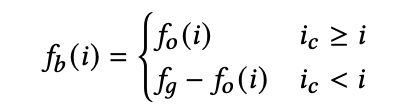This equation asks if the current tick of the pool is below the position’s lower tick. If so, then we know the position cannot be in range[^9]. However, even if the current tick is not below the lower tick, it may still be above the upper tick and thus out of range.

[^9]: This fact is also taken advantage of in the Passive Fee Returns paper. We know that full-range liquidity can by definition never be out of range, so feeGrowthOutside is constant for these ticks. Thus the difference in feeGrowthGlobal from $t_0$ to $t_1$ is the returns for one unit of full-range liquidity.

Next, we must calculate $f_a(i_u)$.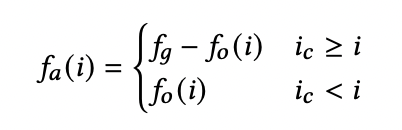This equation asks whether the tick of the pool is above the position’s upper tick and derives the fees above the upper tick.

Both $f_b(i)$ and $f_a(i)$ formulas use the feeGrowthOutside0X128 variable to calculate total fees accumulated above the upper tick and below the lower tick, which we can then subtract from feeGrowthGlobal0X128 to find the total fees accumulated within a position.

### Example position

We’ll use the same USDC/ETH LP position to calculate fees as an example.

| Name | Notation | Found in | Value at the time of writing | | ---------- | ---------- | ---------- | ---------- | | liquidity | $\ell$ | NonfungiblePositionManager | 10860507277202 | | feeGrowthGlobal0X128 | $f_g$ | UniswapV3Pool | 3094836483914812667943230173936420 | | feeGrowthOutside0X128 of the upper tick of the position | $f_o(i_u)$ | UniswapV3Pool | 233371140530963296710329726203514 | feeGrowthOutside0X128 of the lower tick of the position | $f_o(i_l)$ | UniswapV3Pool | 37180414779992829129391081655145 | | feeGrowthInside0LastX128 | $f_r(t_0)$ | NonfungiblePositionManager | 0 | | tickUpper | $i_u$ | NonfungiblePositionManager | 193380 | | tickLower | $i_l$ | NonfungiblePositionManager | 192180 | | tick | $\lfloor i_c \rfloor$ | UniswapV3Pool | 201780 |

To solve for fees in $token_0$ , we must use this equation.

$fees_0 = \ell \ast (f_r(t_1) - f_r(t_0)) / 2^{128}$

We know that $f_r(t_0)=0$ and $\ell=10860507277202$ from the NonfungiblePositionManager smart contract. We can calculate $f_r(t_1)$ by using the values above and formulas mentioned earlier. All of the values above are as of t1 (current time), so we will drop the $t_1$.

$f_r = f_g - f_b(i_l) - f_a(i_u)$

We also know that $f_g = 3094836483914812667943230173936420$ from the Pool Contract. To calculate fees, we just need $f_b(i_1)$ and $f_a(i_u)$.

### Calculate fees below the lower tick$i_c = 201780$ and $i_l = 192180$, which means $201780 \ge 192180$ and the value of $f_o(i_l)$ should be used.

$f_b(i_l) = f_o(i_l) = 37180414779992829129391081655145$

### Calculate fees below the upper tick$i_c=201780$ and $i_u=193380$, so $i_c > i_u$, so $f_a(i_u)=f_g - f_o(i_u)$

$f_a(i_u) = 3094836483914812667943230173936420 -233371140530963296710329726203514$ $f_a(i_u) = 2861465343383849371232900447732906$

We can put both of these together to calculate $f_r$.

$f_r = f_g - f_b(i_l) - f_a(i_u)$ $f_r = 3094836483914812667943230173936420-37180414779992829129391081655145 - f_a(i_u)$ $f_r = 3057656069134819838813839092281275 - 2861465343383849371232900447732906$ $f_r = 196190725750970467580938644548369$

### Putting it all together

$fees_0 = \ell \ast (f_r(t_1) - f_r(t_0)) / 2^{128}$ $fees_0 = 10860507277202 (196190725750970467580938644548369 - 0) / 2^{128}$ $fees_0 = 10860507277202 \ast 5.76552725 \ast 10^{-7}$ $fees_0 = 6261655.06$

$token_0$ is USDC, which has 6 decimal places. Just like we did previously with WETH, to adjust for decimals on $token_0$, we divide by $10^6$.

$adjToken_0 = \frac{6261655.06}{10^6}$ $adjToken_0 = 6.261$

6.261 is the same number the interface gives us for fees for the USDC token!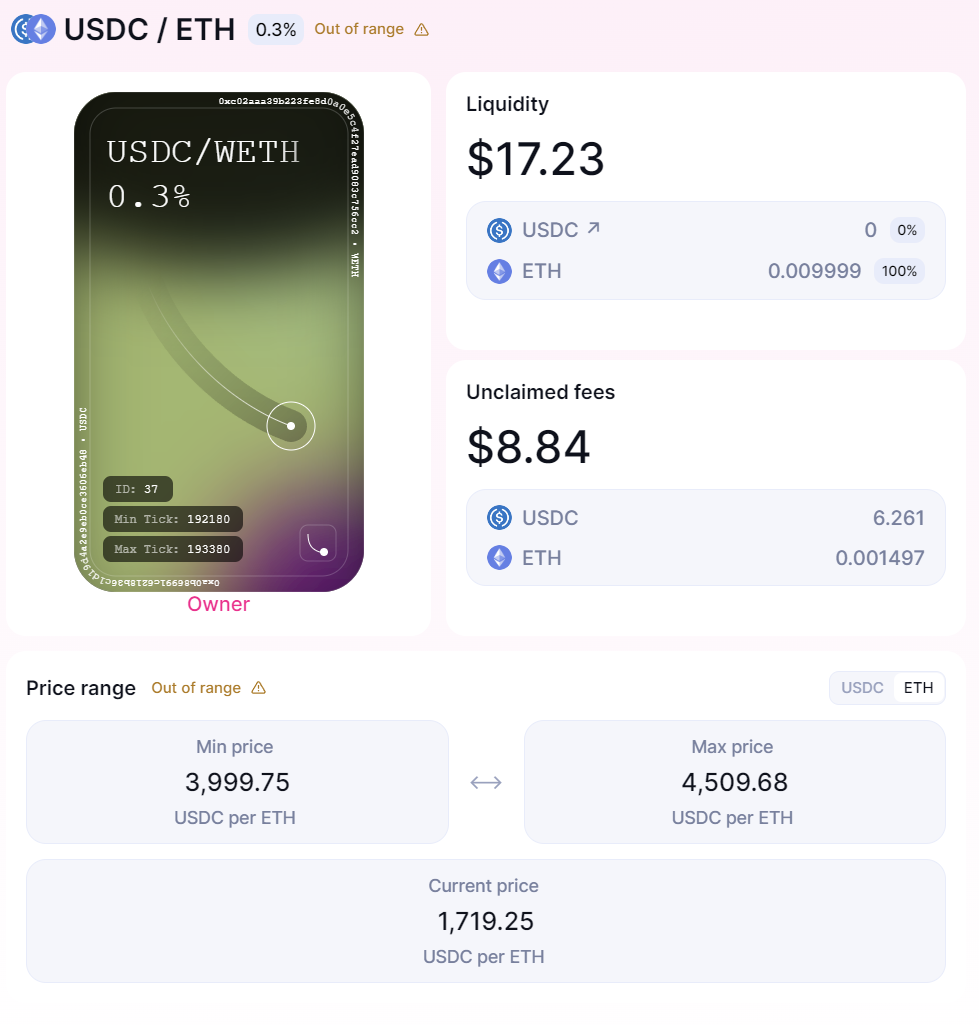## Code example

### Calculating position fees

Getting fees programmatically for any position means querying for the fees above the upper tick, below the lower tick, and the global fee for both tokens in a pool. You’ll also want to retrieve the token decimals to turn the results from being measured in wei to the commonly used number of decimal places.

const ZERO = JSBI.BigInt(0);
const Q128 = JSBI.exponentiate(JSBI.BigInt(2), JSBI.BigInt(128));
const Q256 = JSBI.exponentiate(JSBI.BigInt(2), JSBI.BigInt(256));

// this handles the over and underflows which is needed for all subtraction in the fees math
function subIn256(x, y){
const difference = JSBI.subtract(x, y)
if (JSBI.lessThan(difference, ZERO)) {
} else {
return difference}}

async function getFees(feeGrowthGlobal0, feeGrowthGlobal1, feeGrowth0Low, feeGrowth0Hi, feeGrowthInside0, feeGrowth1Low, feeGrowth1Hi, feeGrowthInside1, liquidity, decimals0, decimals1, tickLower, tickUpper, tickCurrent){
// all needs to be bigNumber
let feeGrowthGlobal_0 = toBigNumber(feeGrowthGlobal0);
let feeGrowthGlobal_1 = toBigNumber(feeGrowthGlobal1);
let tickLowerFeeGrowthOutside_0 = toBigNumber(feeGrowth0Low);
let tickLowerFeeGrowthOutside_1 = toBigNumber(feeGrowth1Low);
let tickUpperFeeGrowthOutside_0 = toBigNumber(feeGrowth0Hi);
let tickUpperFeeGrowthOutside_1 = toBigNumber(feeGrowth1Hi);
// preset variables to 0 BigNumber
let tickLowerFeeGrowthBelow_0 = ZERO;
let tickLowerFeeGrowthBelow_1 = ZERO;
let tickUpperFeeGrowthAbove_0 = ZERO;
let tickUpperFeeGrowthAbove_1 = ZERO;

// As stated above there is different math needed if the position is in or out of range
// If current tick is above the range fg- fo,iu Growth Above range

if (tickCurrent >= tickUpper){
tickUpperFeeGrowthAbove_0 = subIn256(feeGrowthGlobal_0, tickUpperFeeGrowthOutside_0);
tickUpperFeeGrowthAbove_1 = subIn256(feeGrowthGlobal_1, tickUpperFeeGrowthOutside_1);
} else{                // Else if current tick is in range only need fg for upper growth
tickUpperFeeGrowthAbove_0 = tickUpperFeeGrowthOutside_0
tickUpperFeeGrowthAbove_1 = tickUpperFeeGrowthOutside_1
}
// If current tick is in range  only need fg for lower growth
if (tickCurrent >= tickLower){
tickLowerFeeGrowthBelow_0 = tickLowerFeeGrowthOutside_0
tickLowerFeeGrowthBelow_1 = tickLowerFeeGrowthOutside_1
} else{                // If current tick is above the range fg- fo,il Growth below range
tickLowerFeeGrowthBelow_0 = subIn256(feeGrowthGlobal_0, tickLowerFeeGrowthOutside_0);
tickLowerFeeGrowthBelow_1 = subIn256(feeGrowthGlobal_1, tickLowerFeeGrowthOutside_1);
}

//   fr(t1) For both token0 and token1
let fr_t1_0 = subIn256(subIn256(feeGrowthGlobal_0, tickLowerFeeGrowthBelow_0), tickUpperFeeGrowthAbove_0);
let fr_t1_1 = subIn256(subIn256(feeGrowthGlobal_1, tickLowerFeeGrowthBelow_1), tickUpperFeeGrowthAbove_1);
// feeGrowthInside to BigNumber
let feeGrowthInsideLast_0 = toBigNumber(feeGrowthInside0);
let feeGrowthInsideLast_1 = toBigNumber(feeGrowthInside1);

// The final calculations uncollected fees formula
// for both token 0 and token 1 since we now know everything that is needed to compute it
// subtracting the two values and then multiplying with liquidity l *(fr(t1) - fr(t0))
let uncollectedFees_0 = (liquidity * subIn256(fr_t1_0, feeGrowthInsideLast_0)) / Q128;
let uncollectedFees_1 = (liquidity * subIn256(fr_t1_1, feeGrowthInsideLast_1)) / Q128;

console.log("Amount fees token 0 in lowest decimal: "+Math.floor(uncollectedFees_0));
console.log("Amount fees token 1 in lowest decimal: "+Math.floor(uncollectedFees_1));

// Decimal adjustment to get final results
let uncollectedFeesAdjusted_0 = (uncollectedFees_0 / toBigNumber(10**decimals0)).toFixed(decimals0);
let uncollectedFeesAdjusted_1 = (uncollectedFees_1 / toBigNumber(10**decimals1)).toFixed(decimals1);
console.log("Amount fees token 0 Human format: "+uncollectedFeesAdjusted_0);
console.log("Amount fees token 1 Human format: "+uncollectedFeesAdjusted_1);
}

// Output position 1
Amount fees token 0 in lowest decimal: 84250230863135890
Amount fees token 1 in lowest decimal: 661007116889360
Amount fees token 0 Human format: 0.084250230863135891
Amount fees token 1 Human format: 0.000661007116889361


# Conclusion

Overall, we hope that this next chapter of Uniswap v3 math primer is helpful to our users. Helping people gracefully and efficiently access and understand Uniswap v3 and its data will help push the ecosystem to be the best it can be. If there is interest, we would love to dive deeper into specific topics. For now, we hope that this answered the vast majority of questions. For anything else, feel free to join the dev-chat in our discord and ask!